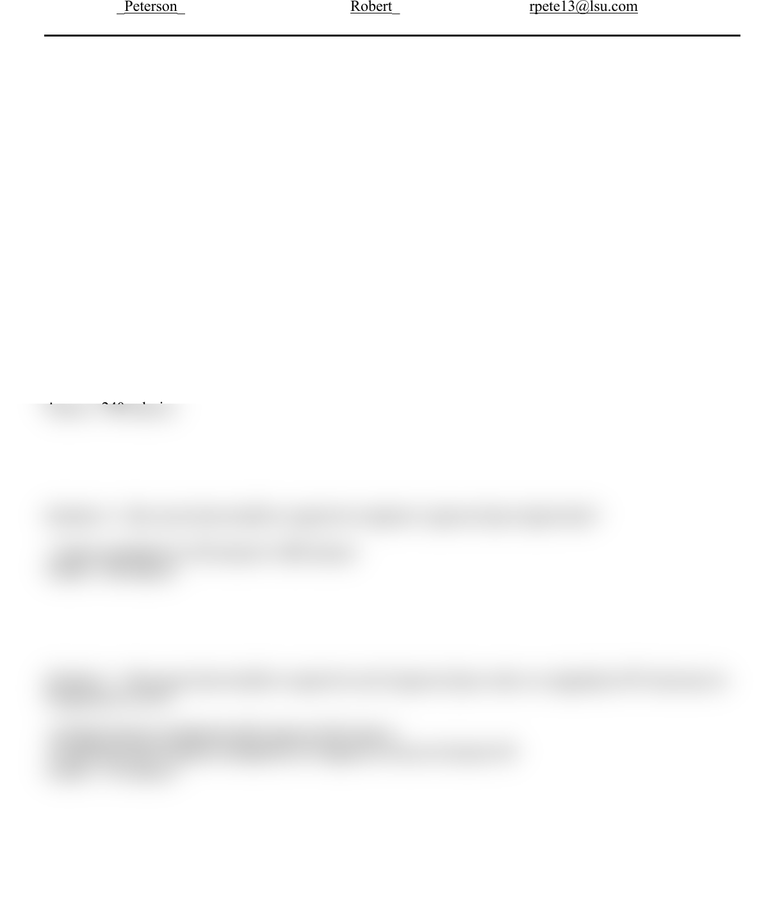Study Guides (380,000)
US (220,000)
LSU (10,000)
HIST (1,000)
HIST 1003 (100)
All (100)
Final

# Terrible History NotesExam

Department
History
Course Code
HIST 1003
Professor
All
Study Guide
Final

This preview shows half of the first page. to view the full 2 pages of the document.HOMEWORK 3 (Due on Monday, September 24, 2012) OCS1005, section 1&2
Last Name:_Peterson__________ First Name:__Robert__________ Email:__rpete13@lsu.com_______
Question 1: How much heat would be required to heat 1 gram of pure liquid water from 10oC to 20oC?
-1 gram multiplied by 10 degrees in terms of calories
Question 2: How much heat would be required to heat 10 grams of pure liquid water from 20oC to 25oC?
-10 grams multiplied by 5 degrees in terms of calories
-50 calories
Question 3: How much heat would be required to melt 3 grams of pure water ice at 0oC and convert it to 3
grams of pure liquid water at 0oC?
-melting=3 grams times 80 calories=240 calories
Question 4: How much heat would be required to evaporate 2 grams of pure liquid water?
-2 grams multiplies by 540 calories=1,080 calories
Question 5: How much heat would be required to melt 3 grams of pure water ice originally at 0oC and raise its
temperature to 10oC?
-melting=3 grams multiplied by 80 calories=240 calories
-temperature raise=3 grams multiplied by 10 degrees in terms of calories=30